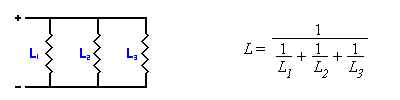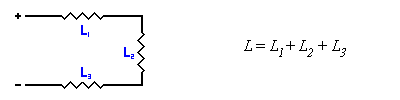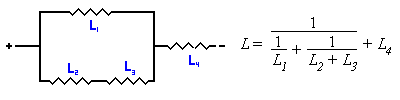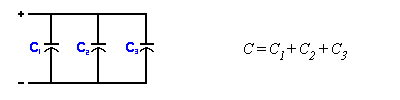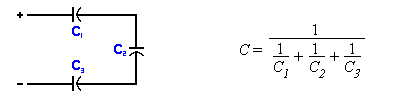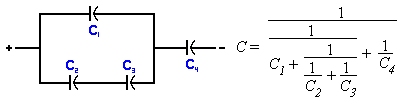# DIY Audio & Video

## Basic Electronics

Here are some basic formulas for wiring capacitors, inductors, and resistors in series or parallel. These are useful when you cannot find a component with the exact value that you are looking for. You can also wire resistors in parallel so that the wattage rating of each resistor only needs to be half (or less) of the total necessary value.

The formulas shown are for inductors (L) and capacitors (C). Resistors (R) act in the same way as inductors, so all inductor formulas can be applied to resistors.